Q1. Write the predecessor of the following numbers.
a) 3,691              b) 10,000          c) 4,999                        d) 6,000
Ans. The predecessors are
a) 3691 ⇒  3690                         b) 10000 ⇒  9999
c) 4999 ⇒  4998                         d) 6000  ⇒  5999

Q2. Write the successor of the following number.
a) 1,050        b) 8,999                        c) 1,00,000                    d) 2,07,080
Ans. The successors are
a) 1,050 ⇒ 1,051                         b) 8,999 ⇒ 9,000
c) 1,00,000 ⇒ 1,00,001            d) 2,07,080 ⇒ 2,07,081

Q3. Do the following additions on the number line.
a) 7 + 3                b) 3 + 5                 c) 1 + 8
Ans.
a) 7 + 3 = 10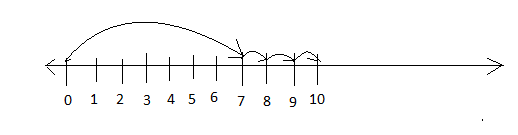b) 3 + 5= 8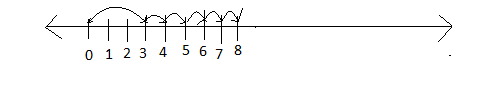c) 1 + 8= 9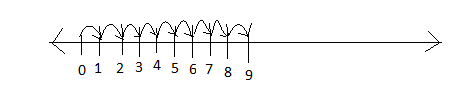Q4. Find the following subtractions on the number line.
a) 7 – 2       b) 9 – 5       c) 8 – 3
Ans.
a) 7−2 = 5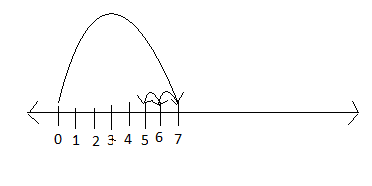b) 9 – 5= 4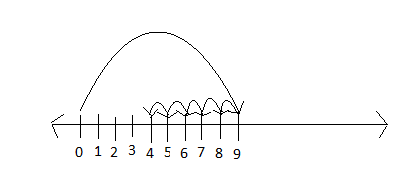c) 8 – 3 = 5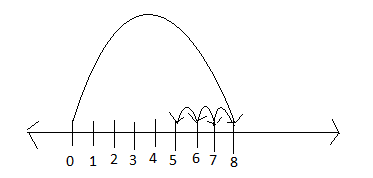Q5.Find the following multiplications on the number line.
a) 2 x 6       b) 3 x 3      c) 4 x 2      d) 5 x 3
Ans.
a)  2 x 6 = 12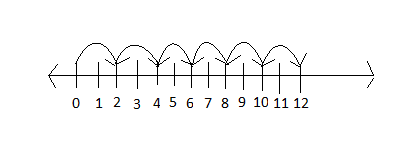b)  3 x 3 = 9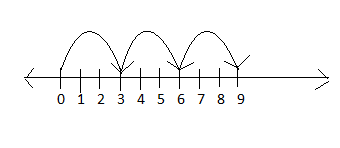c)  4 x 2  = 8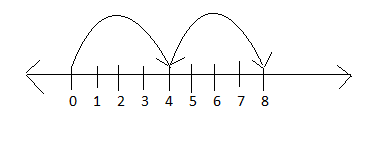d)  5 x 3  = 15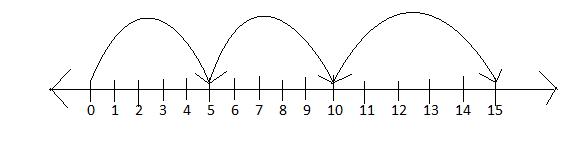Q6. State whether true or false.
1) The whole number 0 has no predecessor
2) Difference between a 3  digit greatest number and a 2  digit smallest number is a 2 digit number.
3) The product of zero and five is five.
4) The difference between 12 and 0 is 0.
5) The sum of a two  digit number  and a four digit number is always a four digit number
6) Any number divided by 0 is not defined.
7) The sum of two whole number is always less their product.
8) 22 + 9 = 9 + 22 represents Associative property.
Ans.
1) True
2) False. Because 999 – 10 = 989 which is a 3  digit number.
3) False. The product of 0 and 5 is zero. 0 x 5 = 0.
4) False. The difference between 12 and 0 is 12.
5) False. Eg 9999 + 99 = 10098 is a five digit number.
6) True.
7)False. Eg 2 + 2 = 4 and 2 x 2 = 4 which is equal.
8) False. It represents commutative property.

Q7. Find the product using distributive property.
a) 35 x 98           b) 42 x 102                c) 17 x 25 + 25 x 4                               d) 8165 x 25 – 8165 x 4.
Ans.
a) 35 x 98
= 35 x (100 – 2)
= (35 x 100) – (35 x 2)
= 3500 – 70
= 3430

b) 42 x 102
= 42 x (100 +2 )
= (42 x 100) + (42 x 2)
= 4200 + 84
= 4284

c) 17 x 25 + 25 x 4= 25 x (17 + 4)
= 25 x 21
= 525

d) 8165 x 25 – 8165 x 4
= 8165 x (25 – 4)
= 8165 x 100
= 816500

Q8. Find the product using suitable rearrangement.
a) 945 x 25 x 4              b) 40 x 228 x 25             c) 25 x 635 x 4
Ans.
a) 945 x 25 x 4
= 945 x 100
= 94500

b) 40 x 228 x 25 = 40 x 25 x 228
= 1000 x 228
= 228000

c) 25 x 635 x 4 = 25 x 4 x 635
= 1000 x 635
= 635000

Q8. Observe the following pattern and extend them by four more terms.
15873 x 7 x 2 = 222222
15873 x 7 x 3 = 333333
15873 x 7 x 4 = 444444
Ans. Next four terms according to the pattern are
15873 x 7 x 5 = 555555
15873 x 7 x 6 = 666666
15873 x 7 x 7 = 777777
15873 x 7 x 8 = 888888

Q9. Observe the pattern and extend by three more terms
1 x 9 + 2 = 11
1 x 9 + 3 = 111
1 x 9 + 4 = 1111
Ans. According to the pattern next three terms are.
1234 x 9 + 5 = 11111
12345 x 9 + 6 = 111111
123456 x 9 + 7 = 1111111

Q10. Mohit buys 15 boxes of chips from one shop and another 30 boxes of chips from another shop. If the cost and number of packets in a box of both types of chips is the same and each chips box costs Rs.250. Find the total money spent on the chips box.
Ans. No of boxes of chips from first shop  = 15
No of boxes of chips from second shop  = 30
Total boxes of chips = (15 + 30)
Cost of one chips box = Rs.250
Therefore, the total cost of chips box = Rs.250 (15 +30)
= Rs.250 x 45
= Rs.11,250
∴ the total money spent on the chips boxes is Rs.11,250.

Q11. An office manager places an order for 85 chairs and 25 tables with a dealer. Each chair costs Rs.160 and each table cost Rs.140. If the manager has given an advance of Rs.6000 to the dealer, then what is the remaining amount to be given?
Ans. Total no. of chairs = 85
Cost of each chair = Rs.160
Therefore, Cost of 85 chairs = (85 x 160)
Total no of tables = 25
Cost of each table = Rs.140
∴  cost of 25 tables = (25 x 140)
∴  total cost of chairs and tables = (85 x 160) + (25 x 140)
= 13,600 + 3,500
= 17,100